TUTORIALS
TUTORIALS HOME

GENERAL MATH
NUMBER SETS
ABSOLUTE VALUE & INEQUALITIES
SETS & INTERVALS
FRACTIONS
POLYNOMIALS
LINEAR EQUATIONS
GEOMETRY
FINITE SERIES
TRIGONOMETRY
EXPONENTS
LOGARITHMS
INDUCTION

CALCULUS
LIMITS
DERIVATIVES
RELATED RATES & OPTIMIZATION
CURVE SKETCHING
INTEGRALS
AREA & VOLUME
INVERSE FUNCTIONS

MAIN
HOME
TESTS
TUTORIALS
SAMPLE PROBLEMS
COMMON MISTAKES
STUDY TIPS
GLOSSARY
CALCULUS APPLICATIONS
MATH HUMOUR

# Series Notation

A sequence is an ordered set of numbers that most often follows some rule (or pattern) to determine the next term in the order. For example, x, x2, x3, x4, ... is a sequence of numbers, where each successive term is multiplied by x.

A series is a summation of the terms of a sequence. The greek letter sigma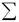is used to represent the summation of terms of a sequence of numbers. Series are typically written in the following form: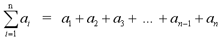where the index of summation, i takes consecutive integer values from the lower limit, 1 to the upper limit, n. The term ai is known as the general term.

A finite series is a summation of a finite number of terms. An infinite series has an infinite number of terms and an upper limit of infinity. This tutorial will deal with finite series. Infinite series will be covered in the calculus tutorials.

# Examples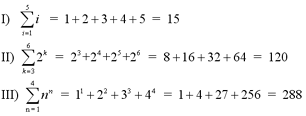# Types of Sequences/Series

There are two main types of sequences. An arithmetic sequence is one in which successive terms differ by the same amount. For example, {3, 6, 9, 12, ...} is an example of an arithmetic sequence, where each term is obtained by adding 3 to the previous term. A geometric sequence is one in which the quotient of any two successive terms is a constant. For example, {3, 9, 27, 81, ...} is an example of a geometric sequence, where each term is obtained by multiplying the previous term by 3. Similarly, there are also arithmetic series and geometric series, which are simply summations of arithmetic and geometric sequences, respectively.

General theorems for arithmetic series and geometric series are listed in the Theorems of Finite Series section below

# Properties of Finite Series

The following are the properties for addition/subtraction and scalar multiplication of series. These properties will help to calculate series whose general term is a polynomial.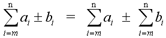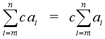# Theorems of Finite Series

The following theorems give formulas to calculate series with common general terms. These formulas, along with the properties listed above, make it possible to solve any series with a polynomial general term, as long as each individual term has a degree of 3 or less.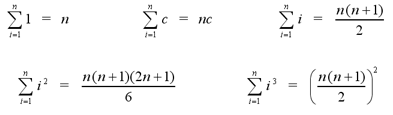• | Proof of finite series theorem with general term i

The following theorem is used for calculating arithmetic series. Suppose we have the following arithmetic series, {a + (a+d) + (a+2d) + ... + (a+(n-1)d}. Then,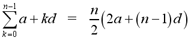The following theorem is used for calculating geometric series. Suppose we have the following geometric series, {a + ar + ar2 + ... + ar(n-1)}. Then,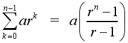• | Proof of geometric series theorem

# Examples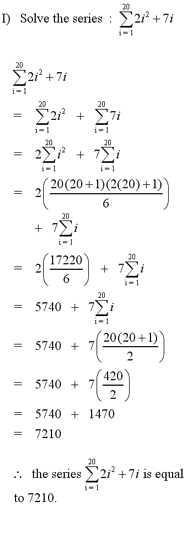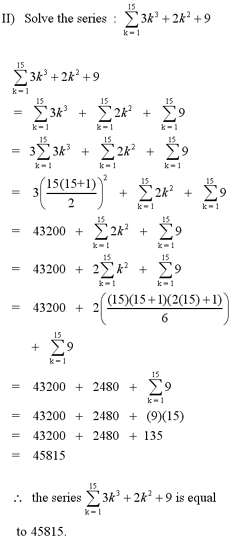Note: For another example of finding the sum of a series, see question #1 in the Additional Examples section at the bottom of the page.

# Telescoping Sums

A telescoping sum is a summation where successive terms cancel each other out. For example,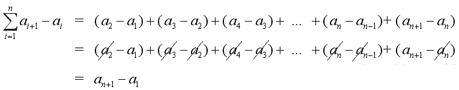is a telescoping sum. This type of series can be easily calculated since all but a few terms are cancelled out.

Note: For an example of a telescoping sums question, see question #2 in the Additional Examples section below.

1 | Determine the sum of the series
2 | Evaluate the telescoping sum
3 | Determine the sum of the geometric series

| Top of Page |

COURSE HOMEPAGES
MATH 1036
MATH 1037

FACULTY HOMEPAGES
Alex Karassev
Ted Chase